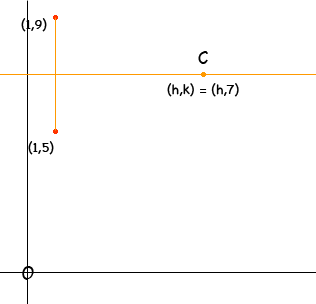Skye Secondary Student hey guys. can you help me with this? Write the equation of a circle having area 15pi and containing the points (1, 5) and (1, 9). so far i have 15 = (x-h)2 + (y-k)2 What do i do next? Skye, Since (1,5) and (1,9) are on the circle they satisfy the equation and hence you can substitute (1,5) and (1,9) into your equation to get two equations in h and k which you can solve. However before attacking any geometry problem I would draw a diagram if possible, and this time a diagram tells me a lot. Since the centre of the circle is equidistant from the two points it must be on the line that is the right bisector of the line segment joining (1,5) and (1,9), that is the line y = 7. Hence k = 7.Penny